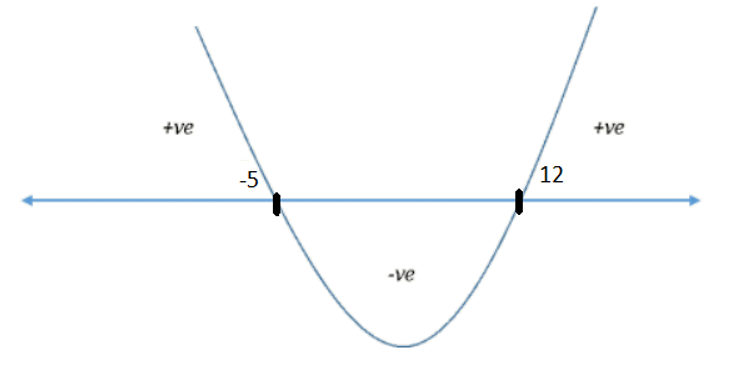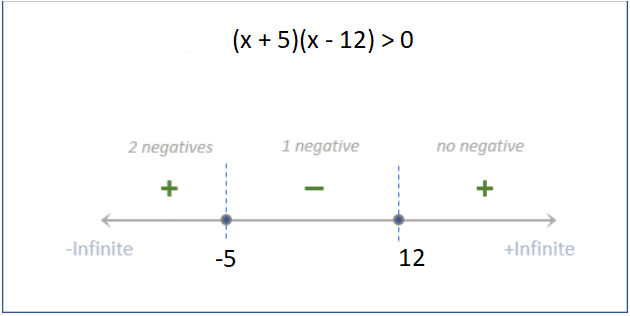GMAT Question of the Day - Daily to your Mailbox; hard ones only

 It is currently 15 Oct 2019, 02:36GMAT Club Daily Prep

Thank you for using the timer - this advanced tool can estimate your performance and suggest more practice questions. We have subscribed you to Daily Prep Questions via email.

Customized
for You

we will pick new questions that match your level based on your Timer History

Track

every week, we’ll send you an estimated GMAT score based on your performance

Practice
Pays

we will pick new questions that match your level based on your Timer History

Not interested in getting valuable practice questions and articles delivered to your email? No problem, unsubscribe here.If |2x - 7| > 17, which of the following must be true ................

 new topic post reply Question banks Downloads My Bookmarks Reviews Important topics
Author Message
TAGS:

Hide Tags

e-GMAT RepresentativeV
Joined: 04 Jan 2015
Posts: 3074
If |2x - 7| > 17, which of the following must be true ................  [#permalink]

Show Tags00:00

Difficulty:15% (low)

Question Stats:79% (01:16) correct21% (01:24) wrongbased on 127 sessions

HideShow timer Statistics

If |2x - 7| > 17, which of the following must be true?

A. -5 < x < 0
B. 0 < x < 12
C. -5 < x < 12
D. x < -5 or x > 12
E. x < -12 or x > 5

To read all our articles: Must read articles to reach Q51

_________________
NUS School ModeratorV
Joined: 18 Jul 2018
Posts: 1024
Location: India
Concentration: Finance, Marketing
WE: Engineering (Energy and Utilities)
Re: If |2x - 7| > 17, which of the following must be true ................  [#permalink]

Show Tags

If |x| > a

Then x > a if x > 0
Or -x > a if x< 0 i.e x < -a

Back to the question
|2x - 7| > 17

2x-7 > 17 If x > 7/2
Or 2x-7 < -17

Solving gives x > 12 or x < -5.

D is the answer.
_________________
Press +1 Kudos If my post helps!
GMAT Club LegendV
Joined: 12 Sep 2015
Posts: 4000
Re: If |2x - 7| > 17, which of the following must be true ................  [#permalink]

Show Tags

Top Contributor
EgmatQuantExpert wrote:
If |2x - 7| > 17, which of the following must be true?

A. -5 < x < 0
B. 0 < x < 12
C. -5 < x < 12
D. x < -5 or x > 12
E. x < -12 or x > 5

----ASIDE-----------------------
When solving inequalities involving ABSOLUTE VALUE, there are 2 things you need to know:
Rule #1: If |something| < k, then –k < something < k
Rule #2: If |something| > k, then EITHER something > k OR something < -k
Note: these rules assume that k is positive

-----ONTO THE QUESTION----------

GIVEN: |2x - 7| > 17
Rule #2 tells us that EITHER 2x - 7 > 17 OR 2x - 7 < -17

Take: 2x - 7 > 17
Add 7 to both sides: 2x > 24
Solve: x > 12

Take: 2x - 7 < -17
Add 7 to both sides: 2x < -10
Solve: x < -5

So, either x > 12 or x < -5

RELATED VIDEO FROM OUR COURSE

_________________
GMAT Club LegendV
Joined: 12 Sep 2015
Posts: 4000
Re: If |2x - 7| > 17, which of the following must be true ................  [#permalink]

Show Tags

Top Contributor
EgmatQuantExpert wrote:
If |2x - 7| > 17, which of the following must be true?

A. -5 < x < 0
B. 0 < x < 12
C. -5 < x < 12
D. x < -5 or x > 12
E. x < -12 or x > 5

Sometimes, the quickest solution to this kind of question involves testing the answer choices

Scan the answer choices
Notice that some answer choices say that x = 1 is a solution, and some say x = 1 is NOT a solution.
So, let's test x = 1
Plug it into the original inequality to get:|2(1) - 7| > 17
Simplify to get: |-5| > 17
NOT true
So, x = 1 is NOT a solution to the inequality.
We can ELIMINATE B and C because they say that x = 1 IS a solution.

Scan the remaining answer choices
Notice that some answer choices say that x = -1 is a solution, and some say x = -1 is NOT a solution.
So, let's test x = -1
Plug it into the original inequality to get:|2(-1) - 7| > 17
Simplify to get: |-9| > 17
NOT true
So, x = -1 is NOT a solution to the inequality.
We can ELIMINATE A because it says x = -1 IS a solution.

We're down to answer choices D or E.
E says x = 6 is a solution, and D says x = 6 is NOT a solution.
So, let's test x = 6
Plug it into the original inequality to get:|2(6) - 7| > 17
Simplify to get: |5| > 17
NOT true
We can ELIMINATE E because it says x = 6 IS a solution.

NOTE: With this particular question, testing the answer choices is a slower approach. However, when solving questions of this nature, the approach is still worth considering, especially with more complex inequalities.

Cheers,
Brent
_________________
e-GMAT RepresentativeV
Joined: 04 Jan 2015
Posts: 3074
Re: If |2x - 7| > 17, which of the following must be true ................  [#permalink]

Show Tags

Solution

Given:
• We are given an inequality, |2x - 7| > 17

To find:
• We need to find out the values of x, which satisfies the given inequality

Approach and Working:
Let’s remove the modulus first, by squaring the inequality on both sides

• Squaring the given inequality on both sides, gives,
o $$(2x – 7)^2 > 17^2$$
o Implies, $$4x^2 – 28x + 49 > 289$$
o $$4x^2 – 28x - 240 > 0$$
o Taking 4 out, we get, $$x^2 – 7x - 60 > 0$$
o Factorising the quadratic expression, we can write the above inequality as,
 (x – 12)(x + 5) > 0

Approach 1: Wavy-line method

The zero points are {-5, 12}, and the wavy-line will be as follows:• The expression will be positive in the regions, x < -5 or x > 12

Approach 2: Number-line method

The zero points are {-5, 12}, and the number-line will be as follows:Therefore, (x – 12)(x + 5) is positive for x < -5 or x > 12

Hence, the correct answer is option D.

_________________Re: If |2x - 7| > 17, which of the following must be true ................   [#permalink] 02 Nov 2018, 04:55
Display posts from previous: Sort by

If |2x - 7| > 17, which of the following must be true ................

 new topic post reply Question banks Downloads My Bookmarks Reviews Important topics

 Powered by phpBB © phpBB Group | Emoji artwork provided by EmojiOne